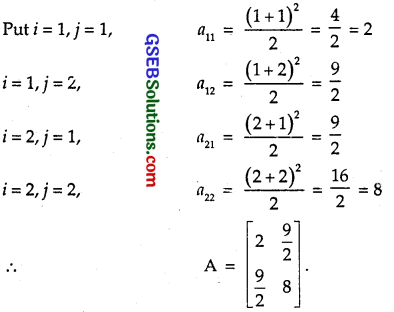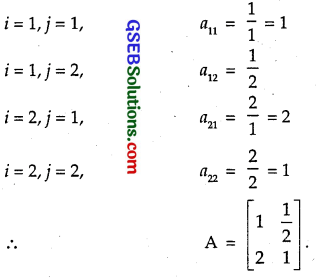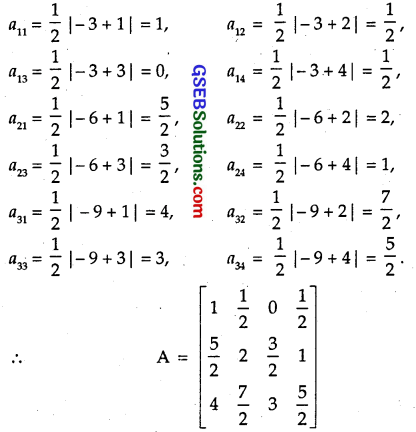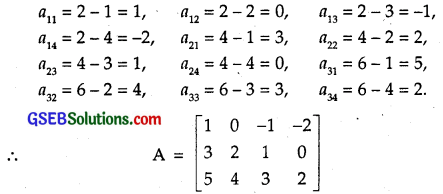# GSEB Solutions Class 12 Maths Chapter 3 Matrices Ex 3.1

Gujarat Board GSEB Textbook Solutions Class 12 Maths Chapter 3 Matrices Ex 3.1 Textbook Questions and Answers.

## Gujarat Board Textbook Solutions Class 12 Maths Chapter 3 Matrices Ex 3.1Question 1.
In the matrix A = $$\left[\begin{array}{cccc} 2 & 5 & 19 & -7 \\ 35 & -2 & \frac{5}{2} & 12 \\ \sqrt{3} & 1 & -5 & 17 \end{array}\right]$$, write :
(i) The order of the matrix.
(ii) The number of elements. .
(iii) Write the elements a13, a21, a33, a24, a23.
Solution:
(i) The order of the matrix = 3 x 4.
(ii) Hie number of elements = 3 x 4 = 12.
(iii) a13 = 19. a21 = 35, a33 = – 5, a24 = 12, a23 = $$\frac { 5 }{ 2 }$$.

Question 2.
If a matrix has 24 elements, what are possible orders it can have? What, if it has 13 elements?
Solution:
(i) 24 = 1 x 24 = 2 x 12 = 3 x 8 = 4 x 6
Thus, there are 8 matrices having 24 elements.
Their orders are 1 x 24, 24 x 1, 2 x 12, 12 x 2, 3 x 8, 8 x 3, 4 x 6, 6 x 4.

(ii) 13 = 1 x 13
There are 2 matrices of 13 elements of orders 1 x 13 and 13 x 1.Question 3.
If a matrix has 18 elements, what are the possible orders it can have? What, if it has 5 elements?
Solution:
(i) 18 = 1 x 18 = 2 x 9 = 3 x 6
There are 6 matrices of orders 1 x 18, 18 x 1, 2 x 9, 9 x 2, 3 x 6, 6 x 3.

(ii) 5 = 1 x 5
There are two matrices with 5 elements of orders 1 x 5 and 5 x 1.

Question 4.
Construct 2 x 2 matrix A = [a] whose elements are given by
(i) aij = $$\frac{(i+j)^{2}}{2}$$
(ii) aij = $$\frac { i }{ j }$$
(iii) aij = $$\frac{(i+2j)^{2}}{2}$$
Solution:
(i) aij = $$\frac{(i+j)^{2}}{2}$$(ii) aij = $$\frac { i }{ j }$$(iii) aij = $$\frac{(i+2j)^{2}}{2}$$Question 5.
Construct a 3 x 4 matrix whose elements are given by:
(i) aij = $$\frac { 1 }{ 2 }$$|-3i+j|
(ii) aij = 2i – j
Solution:
(i) aij = $$\frac { 1 }{ 2 }$$|-3i+j|(ii) aij = 2i – jQuestion 6.
Find the values of x, y and z from the following equations:
(i) $$\left[\begin{array}{ll} 4 & 3 \\ x & 5 \end{array}\right]$$ = $$\left[\begin{array}{ll} y & 3 \\ 1 & 5 \end{array}\right]$$
(ii) $$\left[\begin{array}{ll} x+y & 2 \\ 5+z & xy \end{array}\right]$$ = $$\left[\begin{array}{ll} 6 & 5 \\ 2 & 8 \end{array}\right]$$
(iii) $$\left[\begin{array}{c} x+y+z \\ x+z \\ y+z \end{array}\right]$$ = $$\left[\begin{array}{c} 9 \\ 5 \\ 7 \end{array}\right]$$
Solution:
(i) $$\left[\begin{array}{ll} 4 & 3 \\ x & 5 \end{array}\right]$$ = $$\left[\begin{array}{ll} y & 3 \\ 1 & 5 \end{array}\right]$$
Equating corresponding elements of the two matrices, we get
4 = y, 3 = z, x = 1
i.e.,
x = 1, y = 4, z = 3.

(ii) $$\left[\begin{array}{ll} x+y & 2 \\ 5+z & xy \end{array}\right]$$ = $$\left[\begin{array}{ll} 6 & 5 \\ 2 & 8 \end{array}\right]$$
Comparing the corresponding elements, we get
x + y = 6, 5 + z = 5, xy = 8
∴ y = 6 – x. Putting value of y in xy = 8, we get
x(6 – x) = 8 or x² – 6x + 8 = 0
∴ (x-4)(x-2) = 0 ∴ x = 4, 2
y = 2, 4
Also, 5 + z = 5 ∴ z = 0
∴ x = 4, y = 2, z = 0
or x = 2, y = 4, z = 0

(iii) $$\left[\begin{array}{c} x+y+z \\ x+z \\ y+z \end{array}\right]$$ = $$\left[\begin{array}{c} 9 \\ 5 \\ 7 \end{array}\right]$$
Equating the corresponding elements, we get
x + y + z = 9 … (1)
x + z = 5 … (2)
y + z = 7 … (3)
or (x + y + z) + z = 12
or 9 + z = 12
∴ z = 12 – 9
= 3.
From (2),
x + z = 5
or x + 3 = 5
∴ x = 2.
From (3),
y + z = 7
y + 3 = 7
∴ y = 4.
Thus, x = 2, y = 4, z = 3.
Alternatively : Equation (1) – (2)
y = 4
(1)-(3)
x = 2 [∴ z = 3 [from(1)]]
⇒ x = 2, y = 4, z = 3.Question 7.
Find the values of a, b, c and d from the equation
$$\left[\begin{array}{cc} a-b & 3 a+c \\ 2 a-b & 3 c+d \end{array}\right]$$ = $$\left[\begin{array}{cc} -1 & 5 \\ 0 & 13 \end{array}\right]$$
Solution:
Comparing the corresponding elements of
$$\left[\begin{array}{cc} a-b & 3 a+c \\ 2 a-b & 3 c+d \end{array}\right]$$ = $$\left[\begin{array}{cc} -1 & 5 \\ 0 & 13 \end{array}\right]$$, we get
a – b = – 1, 2a – b = 0,
2a + c – 5, 3c + d = 13
Subtracting a – b = – 1 from 2a – b = 0, we get
a = 1. Therefore, b = 2.
Putting a = 1 in
2a + c = 5, we get
2 + c = 5
∴ c = 3
From
3c + d = 13
9 + d = 13
∴ = 4.
∴ a = 1, b = 2, c = 3, d = 4.

Question 8.
A = [aij]mxn is a square matrix, if
(A) m < n (B) m > n
(C) m-n
(D) None of these.
Solution:
For a square matrix m = n.
∴ Part (C) is the correct answer.

Question 9.
Which of the given values of x and y make the following pair of matrices equal.
$$\left[\begin{array}{cc} 3 x+7 & 5 \\ y+1 & 2-3 x \end{array}\right]$$ = $$\left[\begin{array}{cc} 0 & y-2 \\ 8 & 4 \end{array}\right]$$
(A) x = – $$\frac { 1 }{ 3 }$$, y = 7
(B) Not Possible to find
(C) y = 7, x = – $$\frac { 2 }{ 3 }$$
(D) x = – $$\frac { 1 }{ 3 }$$, y = – $$\frac { 2 }{ 3 }$$
Solution:Here, x has two values viz. – $$\frac { 7 }{ 3 }$$ and – $$\frac { 2 }{ 3 }$$.
∴ Not possible to find values of x and y.
⇒ Part (B) is correct.Question 10.
The number of all possible matrices of order 3 x 3 with each entry 0 or 1 is
(A) 27
(B) 18
(C) 81
(D) 512
Solution:
There are 9 entries in a 3 x 3 matrix. Each place can be filled with 0 or 1.
∴ 9 places can be filled in 29 = 512 ways.
Number of such matrices = 512.
⇒ Part (D) is correct answer.